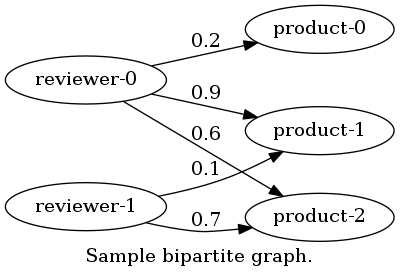# Repeated Improvement Analysis¶

This package provides 4 algorithms for review graph mining project. Mutually Reinforcing Analysis (MRA) algorithm is an algorithm we’ve introduced in DEXA 2011 , Repeated Improvement Analysis (RIA) algorithm is an algorithm we’ve introduced in DEIM 2015 .

Algorithm One has been proposed by Ee-Peng Lim ea al. in CIKM 2010 . Algorithm OneSum is an variation of it made by us.

This package is a part of Review Graph Mining Project which provides other algorithms, datasets, and helper libraries.

## Installation¶

Use pip to install this package.

pip install --upgrade rgmining-ria


## Graph model¶

This package assumes review data are represented in a bipartite graph. This bipartite graph has two kinds of nodes; reviewers and products. One reviewer node and one product node are connected if the reviewer posts a review to the product. In other words, an edge in the graph represents a review. Each review has a rating score. We assume the score is normalized in 0 to 1.

Here is a sample of the bipartite graph.## Usage¶

### Construct a graph¶

This package provides four functions to construct graph objects.

For example, to create a review graph running RIA algorithm,

import ria
graph = ria.ria_graph(1.0)


Then, you need to add reviewer nodes, product nodes, and review edges. new_reviewer() and new_product() methods of the graph create a reviewer node and a product node, respectively, and add them to the graph. Both methods take one argument name, i.e. ID of the node. Note that, the names must be unique in a graph.

add_review() method add a review to the graph. It takes a reviewer, a product, and a normalized rating score which the reviewer posted to the product. The normalized rating scores mean they must be in 0 to 1.

For example, let us assume there are two reviewers and three products like the below.The graph can be constructed by the following code.

# Create reviewers and products.
reviewers = [graph.new_reviewer("reviewer-{0}".format(i)) for i in range(2)]
products = [graph.new_product("product-{0}".format(i)) for i in range(3)]
graph.add_review(reviewers, products, 0.2)
graph.add_review(reviewers, products, 0.9)
graph.add_review(reviewers, products, 0.6)
graph.add_review(reviewers, products, 0.1)
graph.add_review(reviewers, products, 0.7)


### Analysis¶

update() runs one iteration of loopy belief propagation algorithm. This method returns the amount of update in the iteration. You need to run iterations until the amount of update becomes enough small. It’s depended to the review data and the parameter epsilon that how many iterations are required to the amount of update becomes small. Moreover, sometimes it won’t be converged. Thus, you should set some limitation to the iterations.

print("Start iterations.")
max_iteration = 10000
for i in range(max_iteration):

# Run one iteration.
diff = graph.update()
print("Iteration %d ends. (diff=%s)", i + 1, diff)

if diff < 10**-5: # Set 10^-5 as an acceptable small number.
break


### Result¶

Each reviewer has an anomalous score which representing how the reviewer is anomalous. The score is normalized in 0 to 1. To obtain that score, use anomalous_score property.

The ReviewGraph has reviewers property, which returns a collection of reviewers the graph has. Thus, the following code outputs all reviewers’ anomalous score.

for r in graph.reviewers:
print(r.name, r.anomalous_score)


On the other hand, each product has a summarized ratings computed from all reviews posted to the product according to each reviewers’ anomalous score. The summarized ratings are also normalized in 0 to 1. summary property returns such summarized rating.

The ReviewGraph also has products property, which returns a collection of products. Thus, the following code outputs all products’ summarized ratings.

for p in graph.products:
print(p.name, p.summary)


## License¶

This software is released under The GNU General Public License Version 3, see COPYING for more detail.